# Straight Line Depreciation

Refers to a method that when applied to an asset for a year before depreciating it by the same amount each year.

Depreciation is calculated using a straight line technique in which the value of a fixed asset is diminished during its useful life.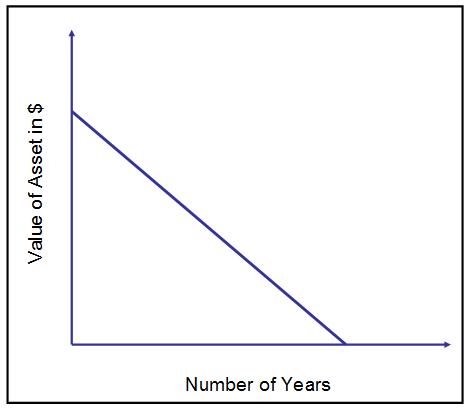Straight line depreciation assumes a constant rate of depreciation. The method is applied to an asset for a year before depreciating it by the same amount each year. A straight line will occur on a graph if the amount of depreciation and the associated period is at a constant rate.

Because the amount of depreciation remains constant from year to year across the useful life of an item, straight-line depreciation is also known as the fixed installment method.

During the life of a fixed asset, an equal amount of value is charged as depreciation in each accounting period to represent the loss value due to wear and tear and obsolescence of the relevant asset.

Depreciation is an accounting value charge that reduces an asset's original cost to its scrap value at the end of its useful life. And this method is the fixed percentage method of the original cost method.

Depreciation is an expense that should be adequately calculated and included in the financial statement.

Most businesses prefer straight-line depreciation, although some use the double declining balancing method or sum of years digit for their books because it results in more write-offs at the beginning of the life of an asset.

For tax calculation purposes, the Internal Revenue Service (IRS) has a depreciation method known as the Modified Accelerated Cost Recovery System (MACRS).

During the first year of use of an asset, the IRS sometimes is not allowed to deduct its full cost. In such a case, the IRS uses MACRS for those assets, including intangible assets, instead of amortizing them.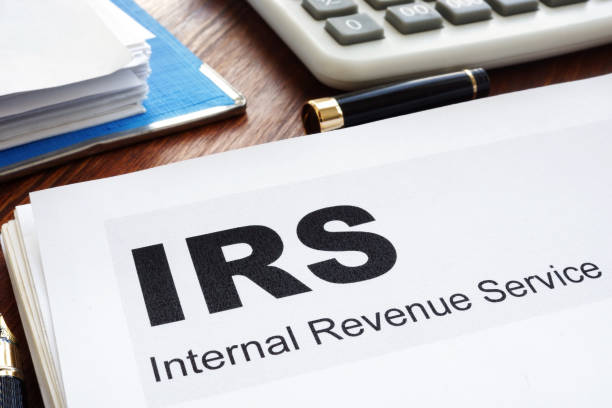For instance, if you are using the straight-line method to calculate depreciation expenses for your company's assets each month. You come across that your total depreciation is \$300.

You then make an entry in your books. You would debit a depreciation expense account of \$300 each month and credit an asset called an accumulated depreciation account.

Journal Entry
Journal EntryDebitCredit
Depreciation Expense\$300
Accumulated Depreciation \$300

These kinds of accounts have a credit balance which implies they decrease the value of an asset as they increase.

## Calculation of the Straight Line Depreciation (SLD)

In this article, three variables are required to calculate the straight-line depreciation of a fixed asset.

1. The Initial Cost (total purchase cost) of an asset (the cost of an asset that includes shipping, taxes, installments, etc.)

2. Scrap or Residual Value of the asset (the price that you think you can sell it for at the end of the month)

3. The Useful Life of an Asset (for many years will it last)

Depreciation = (Cost of an asset - Residual Value / (Estimated useful life of an asset)

In straight-line depreciation, book Value refers to the total value of an asset less accumulated depreciation incurred.

The rate of depreciation under straight-line depreciation is the percentage of the total cost of an asset to be charged as depreciation during the useful lifetime of an asset.

Rate of Depreciation = [1 / (Useful life of an asset)] * 100

Let's take the example of Rajesh, who purchases a tractor and explains it step by step

Step 1: Determine the total cost of a fixed asset

Firstly, you must compute the cost of an asset you're calculating for SLD. The initial cost of an asset will determine how much is depreciated each year.

Let's take the example of a machine to peel bananas for \$8000 plus \$100 for shipping and \$500 for taxes, and have a total of \$8600.

Whenever calculating an asset's cost, ensure to add all related expenses such as labor, material, taxes, and more.

Step 2: Subtract the cost of an asset with the residual value from the amount which is recorded in the books

The residual value is how much you expect an asset to be worth after its useful life. From the above example, a monkey expects the peeling machine to cost \$2000 at the end of its useful life.

So, \$8600 - \$2,000 = \$6,600

Step 3: Determine the useful life of an asset

The type of an asset depends upon the useful life of the asset. In most cases, it is five years and in some seven years.

In this example, we use five years.

Step 4: Calculate the annual rate of depreciation

The annual rate of depreciation is

1 / (Useful life of an asset)

So the rate of depreciation is 1/5 = 0.2

In simple terms, the peeling machine can be depreciated by 20% yearly.

Step 5: Multiply the depreciation rate by the cost of an asset (optional)

In this calculation step, you need to subtract the cost of an asset with residual value. Here we have every value, cost of cost as \$8,600; residual value as \$2000; annual depreciation rate is 20%. Putting it together, we get {(8600-2000) * 20%}, which gives you \$1320.

You can also use another easy way by skipping step 4.

The value is the same, just that instead of the depreciation rate, you fill in the useful life of an asset of 5 years.

Depreciation = (8600 - 2000)/5 = 1320

Hence, the peeling machine depreciation expense is worth \$1320

Step 6: Calculating monthly depreciation

You take the depreciation amount and divide it by 12 to calculate the monthly expense.

1320/12 = 110

The peeling machine monthly will cost you \$110### Everything You Need To Build Your Accounting Skills

To Help You Thrive in the Most Flexible Job in the World.

## How is Straight Line Depreciation (SLD) Different From Other Methods?

There are different methods of depreciation, such as Straight Line Depreciation (SLD), which has been discussed, Double Declining Balancing Method (DDB), Sum of Years Digit Method (SYD), or Unit of Production Method (UPM).

In contrast to the three methods, the straight-line method is the simplest.

For instance, let's take an example of Acer Computer, which buys a \$2,000 McAfee antivirus software that won't be used for five years, and its estimated residual value is \$500.

Let's put the number in the calculation: an asset's total cost is \$2,000, the residual value is \$500, and the estimated lifespan is five years.

Depreciation = (Cost of an asset - Residual Value) / (Estimated Useful Life of an Asset)

Depreciation = (2000 - 500)/5 = 1500/5 =\$300

Here, in SLD, every year, the MacBook depreciates by \$300.

Let's look into the other three methods in the same example.

Straight Line Depreciation (SLD)

For constant five years, \$300 gets depreciated.

Double Declining Balancing Method (DDB)

When using this method, assets depreciate twice as fast as the usual decreasing balance method. It also considers that depreciation charges are higher in the early years of an asset's life and lower in the later years.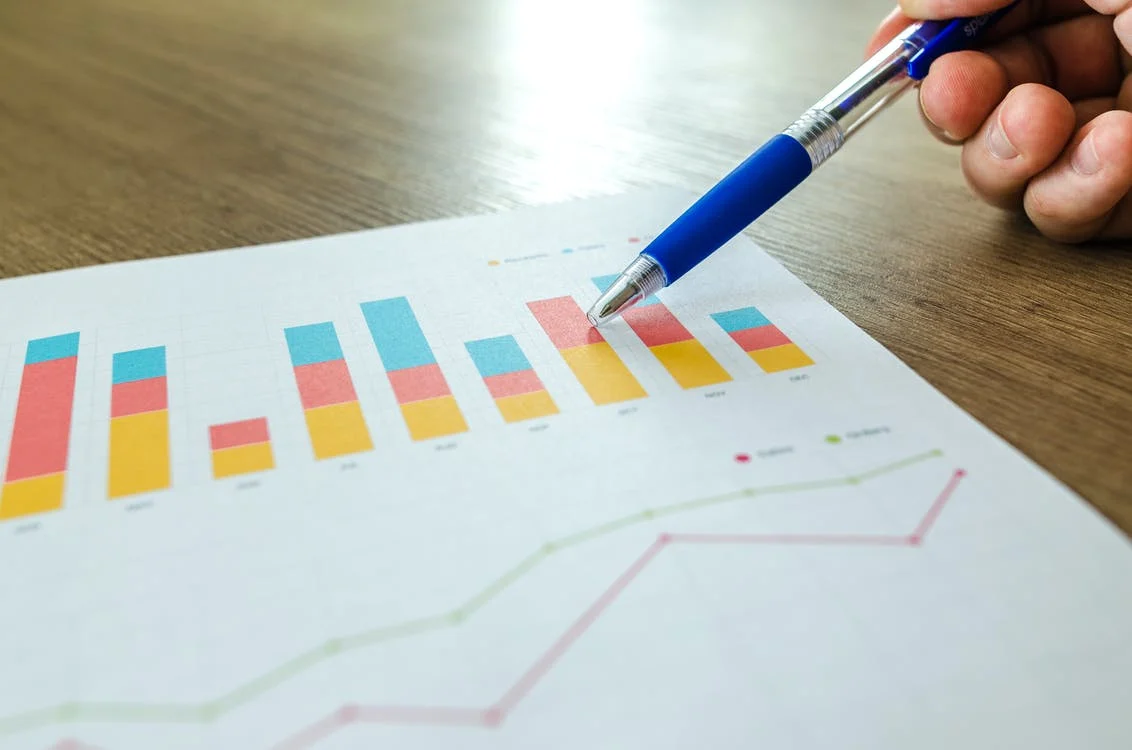The formula used is:

Depreciation = Depreciation Rate(%) * Book Value

Depreciation Rate = 2 * 1 / (Useful Life of an Asset)

Depreciation Rate = 2*1/5 = 0.4%

Applying the formula, here are the decomposition of depreciation and book value:

1st year:

Depreciation: 0.4 * 2000 = 800

Book value: 2000-800=1200;

2nd year:

Depreciation: 0.4 * 1200 = 480;

Book value: 1200-480=720;

3rd year:

Depreciation: 0.4 * 720 = 288;

Book value: 720-288=432;

4th year:

Depreciation: 0.4 * 432 = 173

Book value: 432-173=259;

5th year:

Depreciation: 0.4 * 173 = 69

Book value: 259-69=190

Sum of the Years Digit Method (SYD)

The sum of the years' digits method is an accelerated depreciation that calculates a percentage based on the sum of an asset's useful life years.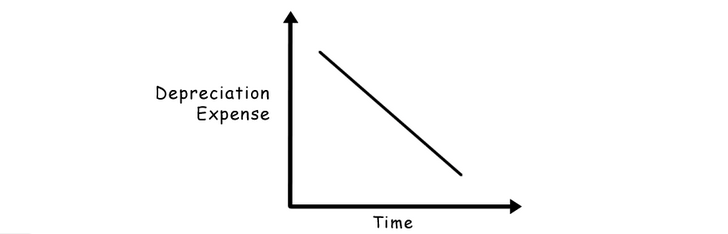The formula used is:

Depreciation = Total Cost of an asset * (Remaining Life of an Asset / Sum of years digit)

Here, the total cost of an asset is 1500; the remaining life of an asset = five years, and the sum of years digits for years  1 + 2 + 3 + 4 + 5 = 15.

So, the total cost of an asset * remaining life of an asset/sum of years digit.

1st year:

1500*5/15=500;

2nd year:

1500*4/15=400;

3rd year:

1500*3/15=300;

4th year:

1500*2/15=200

5th year:

1500*1/15=100.

Table showing three methods of depreciation
Year of DepreciationSLDDDBSYD
1\$300\$800\$500
2\$300\$480\$400
3\$300\$288\$300
4\$300\$173\$200
5\$300\$69\$100

Table showing three methods of depreciation

Notice the three depreciation methods at the start of an asset than at the end.

When it comes to the Unit of production method, it is similar to straight-line depreciation, just that in the straight line method, we measure depreciation using currency. In the Unit of production, we calculate by using units.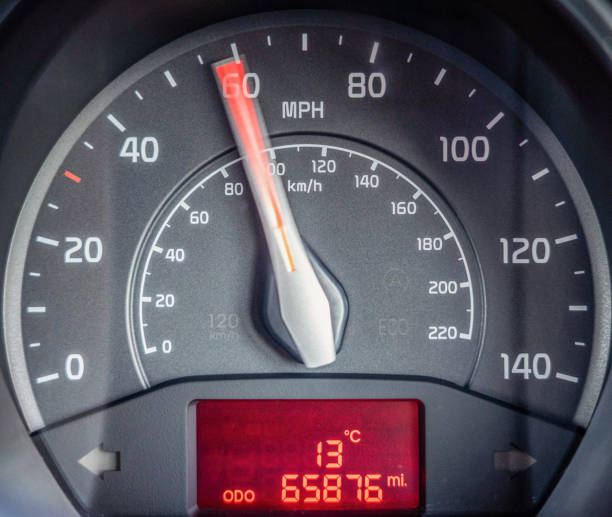The Unit of production can be like the number of km/hr used by a car or the number of miles traveled by airplane, or the number of kWh produced by a power plant.

You'll need two more items for calculating depreciation in production units - the total number of assets produced and the total number of expected production units over a lifetime.

The formula used is:

Depreciation = (Purchase Price - Residual Value * Unit of production) / (Total number of units expected over lifetime)

This method is mainly used for equipment and tools that wear out rather than over time, such as in producing a certain number of units, traveling a certain amount of miles, and producing a certain amount of electricity.

• An asset can be depreciated up to zero in value;

• In the profit and loss account, the equal amount is charged as depreciation every year;

• If there is no scrap value, the book value of an asset can be reduced to zero or the scrap value at the end of its useful life. As a result, the asset account can be written off totally;

• An asset's useful life can be estimated accurately.

The straight-line method is simple and easy to calculate and apply, but it also has some limitations which prevent them from performing.

• In different accounting periods, this method is based on a faulty assumption of the same amount of an asset;

• Each asset acquires a fixed depreciation by applying the predetermined depreciation rate to its original cost. However, the actual usage of the asset is not taken into account while calculating depreciation;

• As time passes by, the work efficiency of an asset decreases, and the repairs and maintenance increase;

• It may be difficult to determine the asset's genuine scrap value after a long period, such as 7 or 15 years from the installation date.### Everything You Need To Master Excel Modeling

To Help You Thrive in the Most Prestigious Jobs on Wall Street.

Researched and authored by Savan Sabu | LinkedIn

Edited by Ka Chun CHIU | LinkedIn